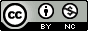## Thursday, February 10, 2011

### Extracting values from R summary objects

This builds on a previous post from Stephen.

I was recently running a series of ANOVA analyses, and I used the aov() function because it had a few options that I preferred. Much like lm(), the function returns an object that you typically pass to summary() to view and interpret the output.

It took me a bit of playing to figure out how to extract the information I needed. My aov object is called "fullmod", and here is the summary() output:

> summary(fullmod)

Error: sample
Df Sum Sq Mean Sq
Type 1 1.4535 1.4535

Error: sample:treatment
Df Sum Sq Mean Sq
treatment 1 0.0086021 0.0086021

Error: Within
Df Sum Sq Mean Sq F value Pr(>F)
treatment 1 0.0077 0.00769 0.0325 0.857505
Type 3 0.3159 0.10529 0.4443 0.722043
age 1 0.0351 0.03512 0.1482 0.701367
sex 1 2.2166 2.21661 9.3547 0.003122 **
hybridization 1 0.1125 0.11255 0.4750 0.492923
Residuals 72 17.0605 0.23695
---
Signif. codes: 0 ‘***’ 0.001 ‘**’ 0.01 ‘*’ 0.05 ‘.’ 0.1 ‘ ’ 1

I assigned the summary output to an object s so I could examine it a bit further.

> s <- summary(fullmod)

Then I peeked under the hood using the str() function.

> str(s)
List of 3
\$ Error: sample :List of 1
..\$ :Classes ‘anova’ and 'data.frame': 1 obs. of 3 variables:
.. ..\$ Df : num 1
.. ..\$ Sum Sq : num 1.45
.. ..\$ Mean Sq: num 1.45
..- attr(*, "class")= chr [1:2] "summary.aov" "listof"
\$ Error: sample:treatment:List of 1
..\$ :Classes ‘anova’ and 'data.frame': 1 obs. of 3 variables:
.. ..\$ Df : num 1
.. ..\$ Sum Sq : num 0.0086
.. ..\$ Mean Sq: num 0.0086
..- attr(*, "class")= chr [1:2] "summary.aov" "listof"
\$ Error: Within :List of 1
..\$ :Classes ‘anova’ and 'data.frame': 6 obs. of 5 variables:
.. ..\$ Df : num [1:6] 1 3 1 1 1 72
.. ..\$ Sum Sq : num [1:6] 0.0077 0.3159 0.0351 2.2166 0.1125 ...
.. ..\$ Mean Sq: num [1:6] 0.0077 0.1053 0.0351 2.2166 0.1125 ...
.. ..\$ F value: num [1:6] 0.0325 0.4443 0.1482 9.3547 0.475 ...
.. ..\$ Pr(>F) : num [1:6] 0.8575 0.72204 0.70137 0.00312 0.49292 ...
..- attr(*, "class")= chr [1:2] "summary.aov" "listof"
- attr(*, "class")= chr "summary.aovlist"

This tells me that s is a list of 3 objects ("Error: sample", "Error: sample:treatment:", and "Error: within"). Each of these objects is a list of 1 element for (some reason!?) with no name or identifier. For "Error: sample" and "Error: sample:treatment:", there are three single numbers. For "Error: Within", each element contains a list of 6 numbers (one for each coefficient in my model).

So, to access the degrees of freedom ("Df") for the "Error: sample", I do the following:

> s[][][]
 1

Or if I prefer to use labels (for the elements that have them)

> s[["Error: sample"]][][["Df"]]
 1

Just to clarify:

s [["Error: sample"]] [] [["Df"]]
^ ^ ^
1st layer 2nd unnamed layer 3rd layer

It is VERY important to use [[ instead of [ here. In the R syntax, [[ retrieves a SINGLE element of the object/array and [ retrieves the full object as a list.

> s["Error: sample"]["Df"]
\$
NULL

See: that didn't work.

To retrieve the p-values I'm interested in, I'll have to dig one more layer deeper to pull the p-value for the coefficient I want (in this case, the 4th element).

> s[][][][]
 0.003122076

OR

> s[["Error: Within"]][][["Pr(>F)"]][]
 0.003122076

Using this basic strategy, you should be able to pull any value from a summary object, but remember that using offsets as I have can be dangerous. For this to work consistently across many models, they must all have the same number of coefficients in the model.

1.Fantastic! Thank you very much!

2.Hello

I was able to extract the proportion of variance from a PCA, so thank you very much.

I would like to use these instead of "PC1" and "PC2" on a plot, but I couldn't figure out how to do it. Could you help me?

3.Great! Thank U from Schiedam!

4.Exactly what I needed, and clear instructions. Thanks!